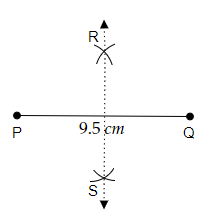# Draw a line segment of length $9.5 \mathrm{~cm}$ and construct its perpendicular bisector.

To do:

We have to draw a line segment of length $9.5\ cm$ and construct its perpendicular bisector.

Solution:Steps of construction:

(i) Let us draw a line segment $\overline{PQ}$ of length $9.5\ cm$.

(ii) Now, with compasses take a measure greater than half of the length of PQ.

(iii) Now, by pointing the pointer of the compasses at point P and point Q respectively. let's draw two arcs above the line segment $\overline{PQ}$ cutting each other and point it as R.

(iv) Similarly, by pointing the pointer of the compasses at point P and point Q respectively. let's draw another two arcs below the line segment $\overline{PQ}$ cutting each other and point it as S.

(iv) Then, let's draw a line segment joining point R and point S.

(v) Therefore, the required perpendicular bisector of the line segment $\overline{PQ}$ is formed.

Updated on: 10-Oct-2022

27 Views